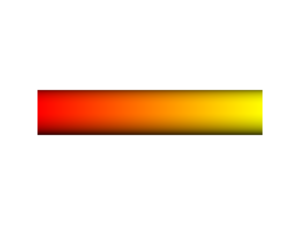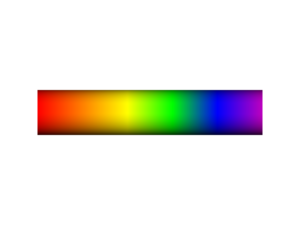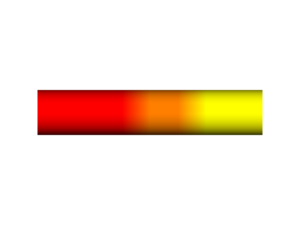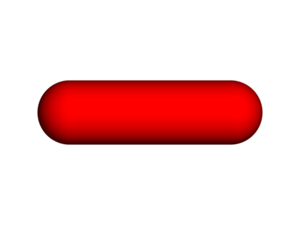# Difference between revisions of "Spectrumbar"

Type Python Script spectrumbar.py Sean M. Law - This code has been put under version control in the project Pymol-script-repo

## Description

Often times one may color their structure based upon some predefined color spectrum. However, this alone is not helpful when dealing with publication images. The purpose of this script is to allow the user to draw their own custom spectrum bar to accompany their structural images.

Further work will be done to improve the functionality of this script. Please feel free to contact the author for function requests.

### USAGE

load the script using the run command

```spectrumbar (RGB_Colors,radius=1.0,name=spectrumbar,head=(0.0,0.0,0.0),tail=(10.0,0.0,0.0),length=10.0, ends=square)
```

Please see the script comments for further custom options. Once the script completes, it will generate a new object called "spectrumbar" (which can be changed through the options) which is a cylinder colored according to the user-specified colors.

Note: It is strongly recommended to turn off the specular reflections before ray tracing

### EXAMPLESFig.1 - spectrumbar redFig.2 - spectrumbar 1,0,0Fig.3 - spectrumbar red, orange, yellowFig.4 - spectrumbar red,orange,yellow,green,blue,purpleFig.5 - spectrumbar 1,0,0, orange, yellow, 0,1,0, 0,0,1, purpleFig.6 - spectrum red,radius=2Fig 7 - spectrumbar red, red, red, orange, yellow, yellowFig 8 - spectrumbar red, radius=2, ends=rounded

## Script

load the script using the run command

```from pymol.cgo import *
from math import *
from pymol import cmd
from re import *

def spectrumbar (*args, **kwargs):

"""
Author Sean M. Law
University of Michigan
seanlaw_(at)_umich_dot_edu

USAGE

While in PyMOL

run spectrumbar.py

Parameter     Preset         Type     Description
RGB_Colors    [1.0,1.0,1.0]  N/A      RGB colors can be specified as a
triplet RGB value or as PyMOL
internal color name (i.e. red)
name          spectrumbar    string   CGO object name for spectrum bar
head          (0.0,0.0,0.0)  float    Starting coordinate for spectrum bar
tail          (10.0,0.0,0.0) float    Ending coordinate for spectrum bar
length        10.0           float    Length of spectrum bar
ends          square         string   For rounded ends use ends=rounded

Examples:

spectrumbar red, green, blue
spectrumbar 1.0,0.0,0.0, 0.0,1.0,0.0, 0.0,0.0,1.0

The above two examples produce the same spectrumbar!

spectrumbar length=20.0

"""

rgb=[1.0, 1.0, 1.0]
name="spectrumbar"
ends="square"
x1=0
y1=0
z1=0
x2=10
y2=0
z2=0
num=re.compile('[0-9]')
abc=re.compile('[a-z]')

for key in kwargs:
elif (key == "name"):
name=kwargs["name"]
elif (key == "tail"):
tail=kwargs["tail"]
tail=tail.strip('" []()')
x2,y2,z2=map(float,tail.split(','))
elif (key == "length"):
if (abc.search(kwargs["length"])):
print "Error: The length must be a value"
return
else:
x2=float(kwargs["length"]);
elif (key == "ends"):
ends=kwargs["ends"]
elif (key != "_self"):
print "Ignoring unknown option \""+key+"\""
else:
continue

args=list(args)
if (len(args)>=1):
rgb=[]
while (len(args)>=1):
if (num.search(args) and abc.search(args)):
if (str(cmd.get_color_tuple(args)) != "None"):
rgb.extend(cmd.get_color_tuple(args.pop(0)))
else:
return
elif (num.search(args)):
rgb.extend([float(args.pop(0))])
elif (abc.search(args)):
if (str(cmd.get_color_tuple(args)) != "None"):
rgb.extend(cmd.get_color_tuple(args.pop(0)))
else:
return
else:
print "Error: Unrecognized color format \""+args+"\""
return

if (len(rgb) % 3):
print "Error: Missing RGB value"
print "Please double check RGB values"
return

dx=x2-x1
dy=y2-y1
dz=z2-z1
if (len(rgb) == 3):
rgb.extend([rgb])
rgb.extend([rgb])
rgb.extend([rgb])
t=1.0/(len(rgb)/3.0-1)
c=len(rgb)/3-1
s=0
bar=[]

while (s < c):
if (len(rgb) >0):
r=rgb.pop(0)
g=rgb.pop(0)
b=rgb.pop(0)
if (s == 0 and ends == "rounded"):
bar.extend([COLOR, float(r), float(g), float(b), SPHERE, x1+(s*t)*dx, y1+(s*t)*dy, z1+(s*t)*dz, radius])
bar.extend([CYLINDER])
bar.extend([x1+(s*t)*dx, y1+(s*t)*dy, z1+(s*t)*dz])
bar.extend([x1+(s+1)*t*dx, y1+(s+1)*t*dy, z1+(s+1)*t*dz])
if (len(rgb) >= 3):
bar.extend([float(rgb), float(rgb), float(rgb)])
r=rgb
g=rgb
b=rgb
else:
bar.extend([float(r), float(g), float(b)])
if (s == c-1 and ends == "rounded"):
bar.extend([COLOR, float(r), float(g), float(b), SPHERE, x1+(s+1)*t*dx, y1+(s+1)*t*dy, z1+(s+1)*t*dz, radius])
s=s+1

cmd.delete(name)